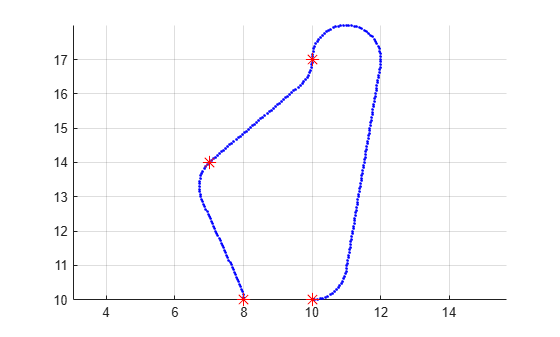# distance

Estimate cost of propagating to target state

Since R2021b

## Syntax

``h = distance(mobileProp,q1,q2)``

## Description

example

````h = distance(mobileProp,q1,q2)` estimates the cost of propagating from one state to another. The `DistanceEstimator` property of the state propagator defines the distance metric for approximating cost.```

## Examples

collapse all

Create a state propagator and specify the distance metric for estimating propagation cost.

`propagator = mobileRobotPropagator(DistanceEstimator="dubins");`

Create a Dubins state space.

`dubinsSpace = stateSpaceDubins([0 25; 0 25; -pi pi]);`

Update the state space of the state propagator using the created state space.

```propagator.StateSpace = dubinsSpace; setup(propagator)```

Create a `navPath` object based on multiple waypoints in a Dubins space.

```path = navPath(dubinsSpace); waypoints = [8 10 pi/2; 7 14 pi/4; 10 17 pi/2; 10 10 -pi]; append(path,waypoints)```

Interpolate that path so that it contains exactly 250 points.

```numStates = 250; interpolate(path,numStates)```

Extract the sequence of motions from the path.

```q1 = path.States(1:end-1,:); % Initial states q2 = path.States(2:end,:); % Final states```

Estimate the cost of propagating to target state.

`cost = distance(propagator,q1,q2);`

Generate a series of control commands and number of steps to move from the current state `q1` with control command `u` toward the target state `q2`.

```u = zeros(size(q1,1),propagator.NumControlOutput); steps = zeros(size(q1,1),1); for i = 1:size(q1,1) [u(i+1,:),steps(i)] = sampleControl(propagator,q1(i,:),u(i,:),q2(i,:)); end```

Create a control-based path object with the specified state propagator and a sequence of specified states, controls, targets, and durations.

```states = path.States; controls = u(2:end,:); targets = q2; durations = steps*propagator.ControlStepSize; path2 = navPathControl(propagator,states,controls,targets,durations);```

Visualize the results.

```figure grid on axis equal hold on plot(path2.States(:,1),path2.States(:,2),".b") plot(waypoints(:,1),waypoints(:,2),"*r","MarkerSize",10)```## Input Arguments

collapse all

Mobile robot state propagator, specified as a `mobileRobotPropagator` object.

Initial states, specified as an n-by-s matrix. n is the number of states and s is the size of the state vector.

Final states, specified as an n-by-s matrix. n is the number of states and s is the size of the state vector.

## Output Arguments

collapse all

Cost values, returned as an n-element vector. n is the number of `q1` and `q2` pairs.

You can use the cost values returned by this function to find the nearest neighbor for sampled target states when planning a path.

## Version History

Introduced in R2021b